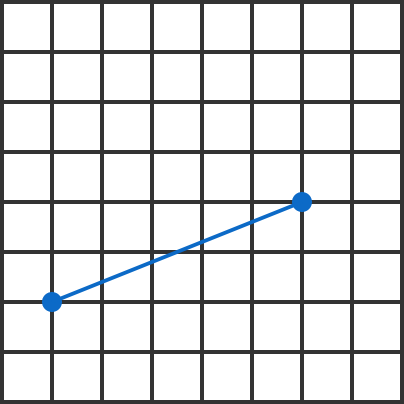# Don't Cross A Corner!On an $n \times n$ square grid, two vertices are chosen at random and a segment is drawn connecting them. As $n\rightarrow \infty,$ what is the probability that the segment does not cross any vertices of this graph besides the two chosen ones?

×